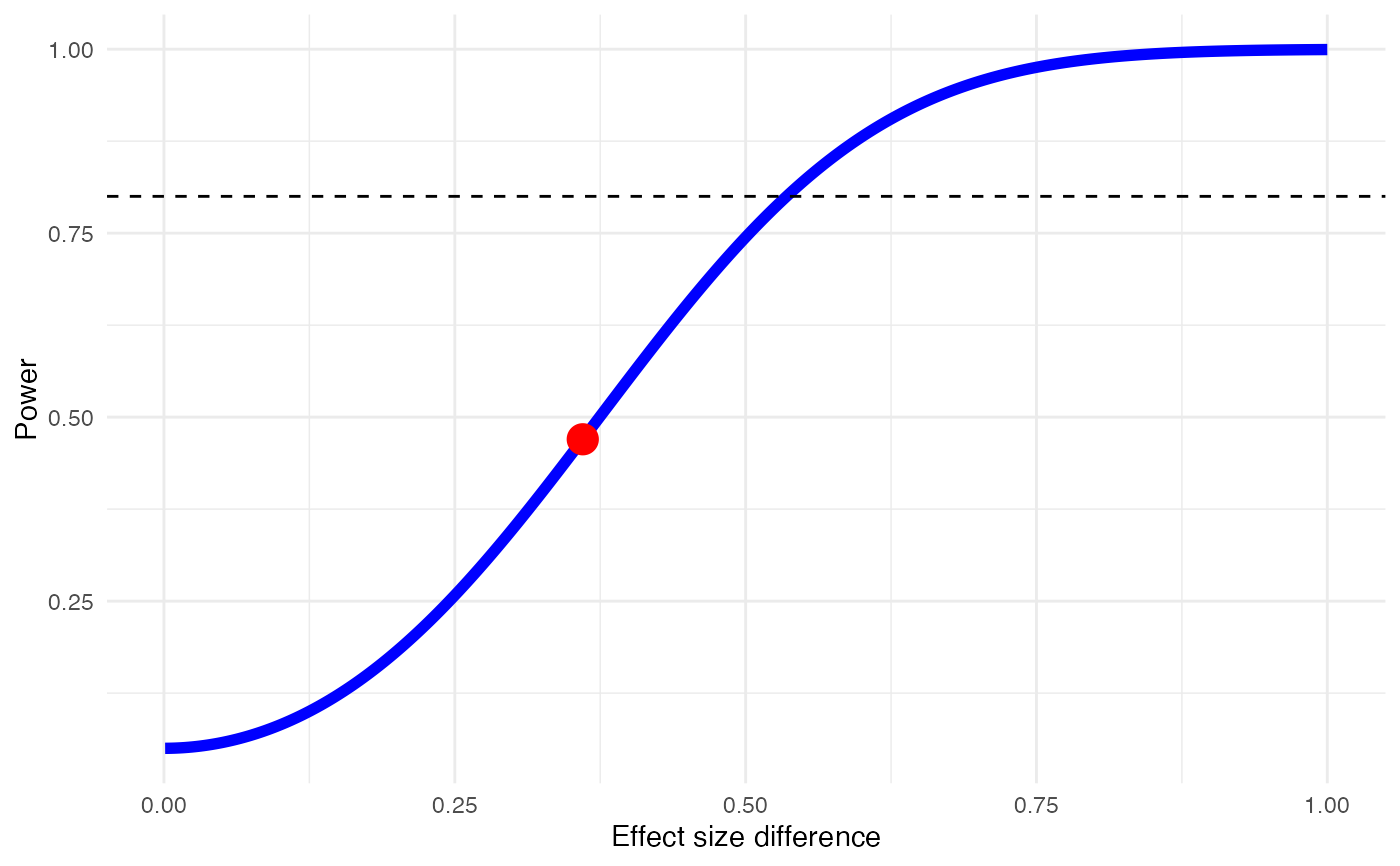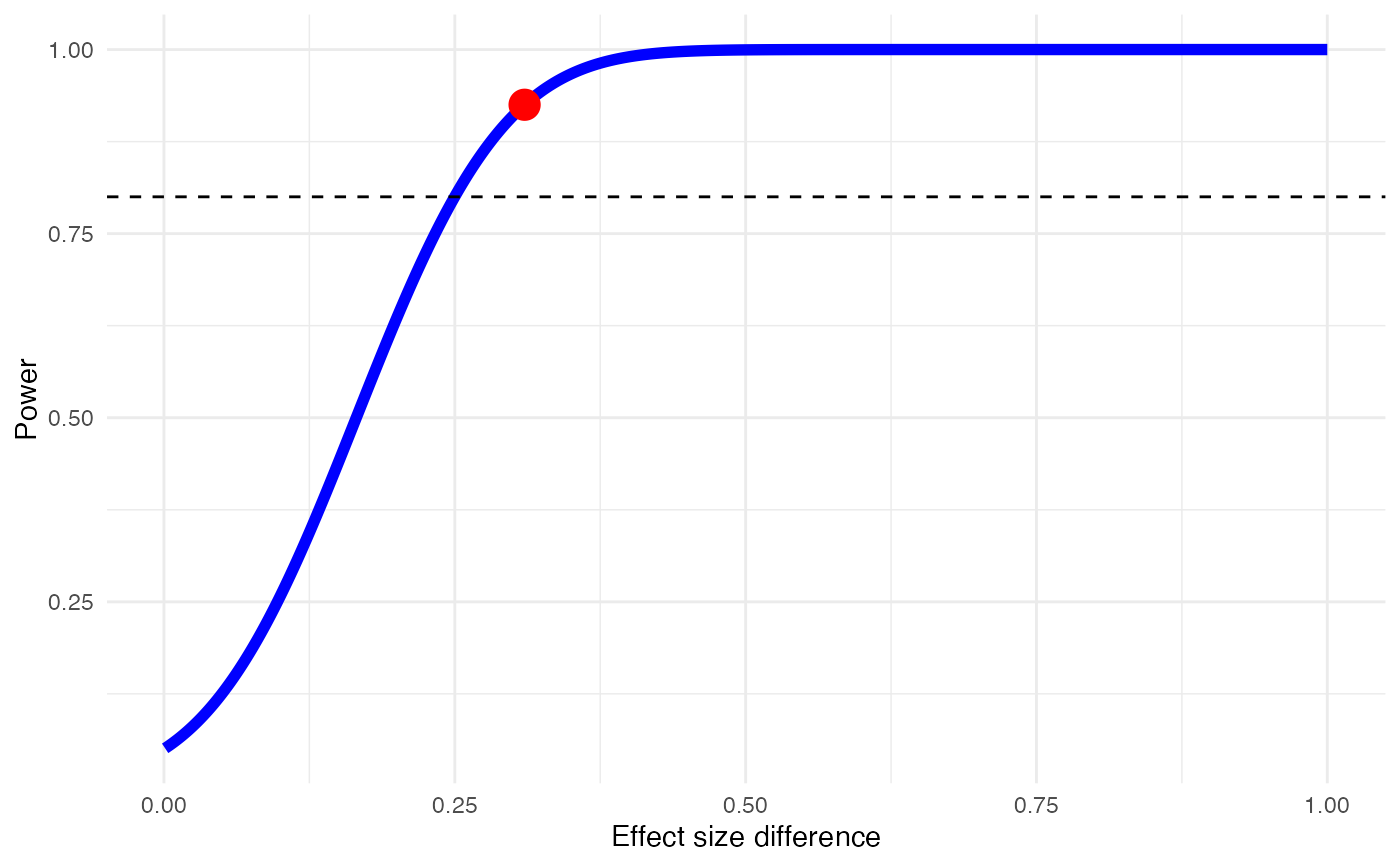This function performs an a priori power estimation for a test for subgroup differences within a meta-analysis.

power.analysis.subgroup(TE1, TE2, seTE1, seTE2, sd1, sd2, var1, var2,
two.tailed=TRUE)

## Arguments

TE1 Pooled effect size (e.g., standardized mean difference, Hedges' $$g$$, log-Odds Ratio or other linear continuous effect size) of the first subgroup of studies. Pooled effect size (e.g., standardized mean difference, Hedges' $$g$$, log-Odds Ratio or other linear continuous effect size) of the second subgroup of studies. Pooled standard error of the first subgroup of studies. Either seTE1/seTE2, sd1/sd2, or var1/var2 must be provided. Pooled standard error of the second subgroup of studies. Either seTE1/seTE2, sd1/sd2, or var1/var2 must be provided. Pooled standard deviation of the first subgroup of studies. Either seTE1/seTE2, sd1/sd2, or var1/var2 must be provided. Pooled standard deviation of the second subgroup of studies. Either seTE1/seTE2, sd1/sd2, or var1/var2 must be provided. Pooled variance of the first subgroup of studies. Either seTE1/seTE2, sd1/sd2, or var1/var2 must be provided. Pooled variance of the second subgroup of studies. Either seTE1/seTE2, sd1/sd2, or var1/var2 must be provided. Logical. Should a two-tailed (TRUE) or one-tailed (FALSE) test ($$\alpha = 0.05$$) be assumed? Default is TRUE.

## Value

Returns a list with five elements:

• Power: The estimated power of the subgroup contrast, expressed as a value between 0 and 1 (i.e., 0%-100%).

• Plot: A plot showing the effect size difference (x), power (y), estimated power (red point) and estimated power for changing effect size differences (blue line). A dashed line at 80% power is also provided as a visual threshold for sufficient power.

• Data: A data.frame containing the data used to generate the plot in Plot.

• Test: The type of test used for the power calculations ("one.tailed" or "two.tailed").

• Gamma: The analyzed effect size difference calculated from the inputs.

## Details

This function provides an estimate of the power $$1-\beta$$ of a subgroup contrast analysis provided the assumed effect sizes in each subgroup and their dispersion measures. The function implements the formulae described by Hedges and Pigott (2001).

Hedges, L. V., & Pigott, T. D. (2001). The power of statistical tests in meta-analysis. Psychological methods, 6(3), 203.

power.analysis

## Examples

# Example 1: using standard error and two-tailed test
power.analysis.subgroup(TE1=0.30, TE2=0.66, seTE1=0.13, seTE2=0.14)#> Minimum effect size difference needed for sufficient power: 0.536 (input: 0.36)
#> Power for subgroup difference test (two-tailed): 46.99%# Example 2: using variance and one-tailed test
pasg = power.analysis.subgroup(TE1=-0.91, TE2=-1.22, var1 = 0.0023, var2 = 0.0078,
two.tailed = FALSE)
summary(pasg)#> Minimum effect size difference needed for sufficient power: 0.25 (input: 0.31)
#> Power for subgroup difference test (one-tailed): 92.5%# Only show plot
plot(pasg)